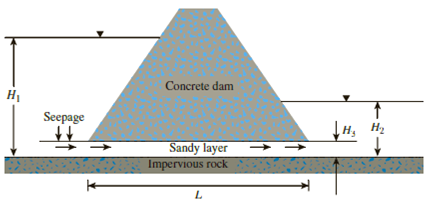Chapter 7, Problem 7.3PPrinciples of Geotechnical Enginee...

9th Edition
Braja M. Das + 1 other
ISBN: 9781305970939

Solutions

Chapter
SectionPrinciples of Geotechnical Enginee...

9th Edition
Braja M. Das + 1 other
ISBN: 9781305970939
Textbook Problem

Seepage is occurring through the sandy layer underneath the concrete dam as shown in Figure 7.30.Figure 7.30Given: upstream water level, H1 = 16 m; downstream water level, H2 = 2.3 m; thickness of the sandy layer, H3 = 0.75 m; hydraulic conductivity of the sandy layer, k = 0.009 cm/sec; void ratio of sand, e = 0.8; and L = 45 m. Determine:a. Rate of seepage per unit length of the dam (in m3/hr/m)b. Seepage velocityc. Quantity of seepage per day if the dam is 350 m long

(a)

To determine

The rate of seepage per unit length of the dam.

Explanation

Given information:

The upstream water level H1 is 16 m.

The downstream water level H2 is 2.3 m.

The thickness of the sandy layer H3 is 0.75 m.

The hydraulic conductivity of the sandy layer k is 0.009 cm/sec.

The void ratio of sand e is 0.8.

The value of length L is 45 m.

Calculation:

Determine the hydraulic gradient using the relation.

i=ΔhL=H1H2L (1)

Substitute 16 m for H1, 2.3 m for H2, 45 m for L.

i=162.345=0.304

Determine the rate of seepage per unit length of the dam using the relation.

q=kiA

Here, A is the area of the sandy layer

(b)

To determine

The seepage velocity.

(c)

To determine

The quantity of seepage per day for 350 m long dam.

Still sussing out bartleby?

Check out a sample textbook solution.

See a sample solution

The Solution to Your Study Problems

Bartleby provides explanations to thousands of textbook problems written by our experts, many with advanced degrees!

Get Started